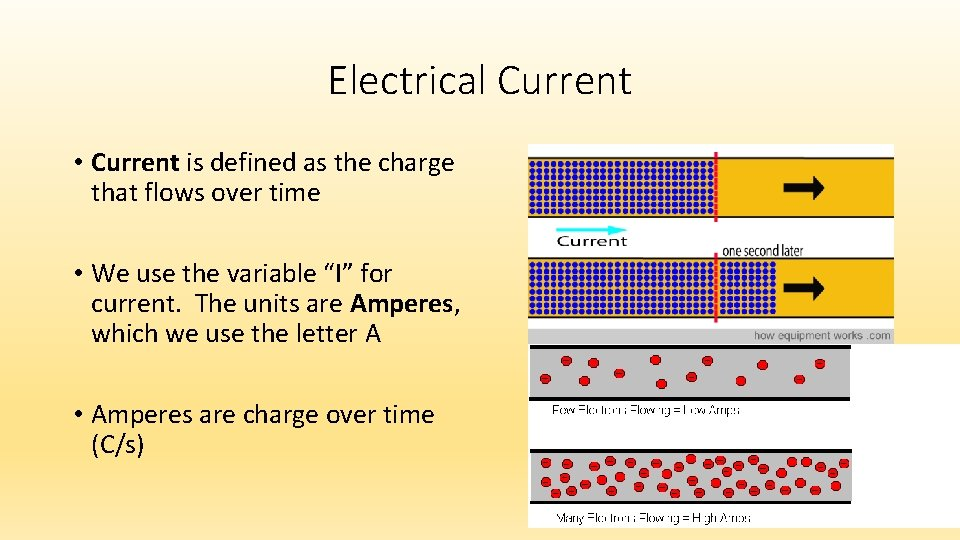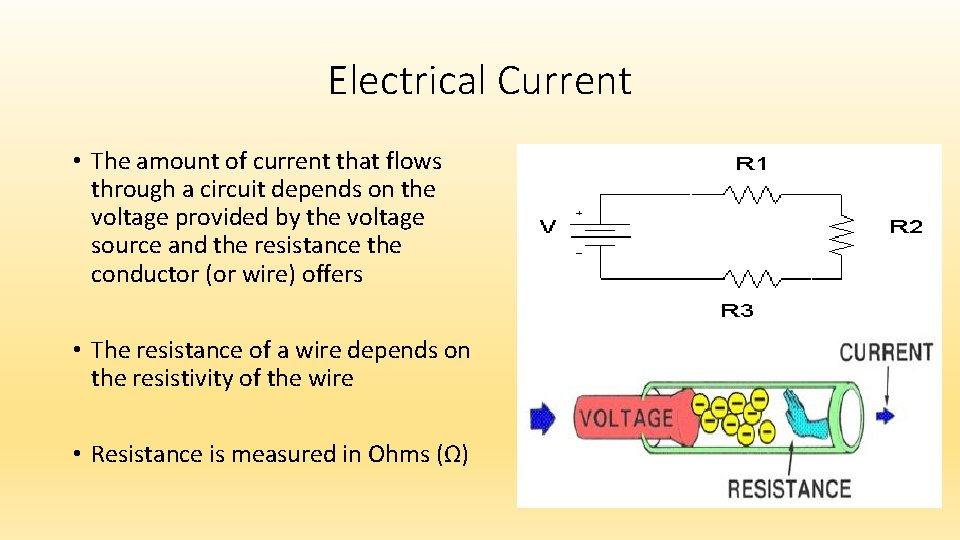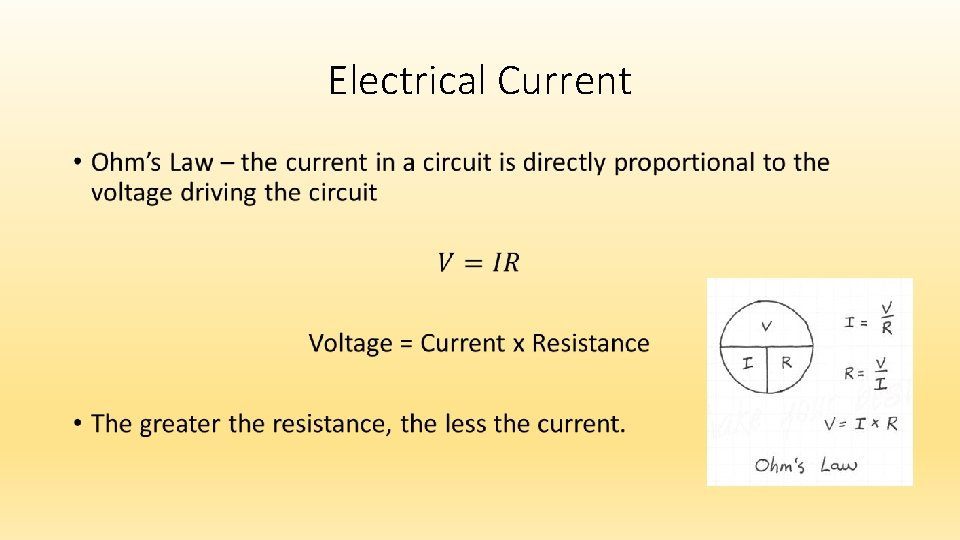# Electric Current Electrical Current Current is defined as

• Slides: 9Electric CurrentElectrical Current • Current is defined as the charge that flows over time • We use the variable “I” for current. The units are Amperes, which we use the letter A • Amperes are charge over time (C/s)Electrical Current • Charge flows from one place to another when there is a potential difference, or difference in the voltage between the ends of a conductor • Charge will continue to flow until there is no difference between the potentials on the conductorsElectrical Current • Electric charges flow from high potential to low potential • Just like mass moves from high potential to low potential • Just like fluids move from high pressure to low pressureElectrical Current • In solid conductors electrons carry the charge for current as they are allowed to move freely through the conductor. • In fluids, both the positive and negative ions as well as electrons move as current.Electrical Current • Something that provides a potential difference is a voltage source. • Batteries and generators are capable of maintaining a steady flow of charges as they move from high to low potential. • Batteries supply the energy that allows charges to move.Electrical Current • The amount of current that flows through a circuit depends on the voltage provided by the voltage source and the resistance the conductor (or wire) offers • The resistance of a wire depends on the resistivity of the wire • Resistance is measured in Ohms (Ω)Electrical Current •Electrical Current • Worksheet • Then go to page 545 # 5, 7, 12, 26, 28